Next: The dendrite Up: Dendrites Previous: Universal dendrites of order

## Other universal dendrites

For a given nonempty set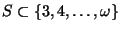we denote by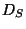any dendrite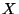satisfying the following two conditions:

(a)
if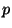is a ramification point of, then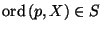;
(b)
for each arc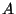contained inand for every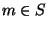there is ina pointwith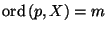.
It is shown in [Charatonik W.J. et al. 1994, Theorem 6.2, p. 229] that the dendriteis topologically unique, i.e., if two dendrites satisfy conditions (a) and (b) with the same set, then they are homeomorphic. The dendriteis called the standard universal dendrite of orders in. Ifis a singleton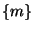, thenis just the standard universal dendrite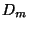defined previously.

The following properties of dendritesare known (see [Charatonik W.J. et al. 1994, Theorems 6.4 and 6.6-6.8, p. 230; Corollary 6.10, p. 232]).

1. For any nonempty subset, the dendriteis strongly pointwise self-homeomorphic.
2. If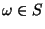, then the dendriteis universal for the family of all dendrites.
3. If the setis finite with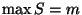, thenis universal for the family of all dendrites having orders of ramification points at most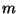.
4. If the setis infinite and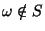, thenis universal for the family of all dendrites having finite orders of ramification points.
5. Nonconstant open images of standard universal dendritesare homeomorphic toif and only ifis a nonempty subset of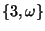.
6. For any nonempty subsetand for an arbitrary dendrite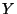there exists a monotone mapping fromonto, [Charatonik et al. 1998, Theorem 2.22, p. 239].
7. For any nonempty subsetthe dendriteis monotonely homogeneous, [Charatonik 1996, Theorem 3.3, p. 292].

Here you can find source files of this example.

Here you can check the table of properties of individual continua.

Here you can read Notes or write to Notes ies of individual continua.Next: The dendrite Up: Dendrites Previous: Universal dendrites of order
Janusz J. Charatonik, Pawel Krupski and Pavel Pyrih
2001-11-30